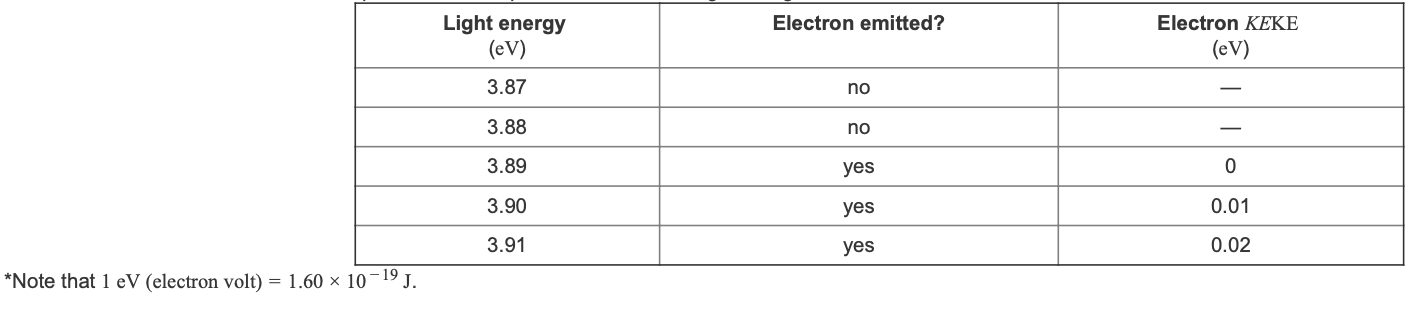# Problem: Part C. What is the kinetic energy of the emitted electrons when cesium is exposed to UV rays of frequency 1.80 × 1015 Hz?Express your answer in joules to three significant figures.Electrons are emitted from the surface of a metal when it's exposed to light. This is called the photoelectric effect. Each metal has a certain threshold frequency of light, below which nothing happens. Right at this threshold frequency, an electron is emitted. Above this frequency, the electron is emitted and the extra energy is transferred to the electron.The equation for this phenomenon isKE = hν − hν0where KE is the kinetic energy of the emitted electron, h = 6.63 × 10−34 J•s is Planck's constant, ν is the frequency of the light, and ν0 is the threshold frequency of the metal.Also, since E = hν, the equation can also be written asKE = E − φwhere E is the energy of the light and φ is the binding energy of the electron in the metal.Here are some data collected on a sample of cesium exposed to various energies of light.

###### FREE Expert SolutionView Complete Written Solution
###### Problem Details

Part C. What is the kinetic energy of the emitted electrons when cesium is exposed to UV rays of frequency 1.80 × 1015 Hz?

Electrons are emitted from the surface of a metal when it's exposed to light. This is called the photoelectric effect. Each metal has a certain threshold frequency of light, below which nothing happens. Right at this threshold frequency, an electron is emitted. Above this frequency, the electron is emitted and the extra energy is transferred to the electron.

The equation for this phenomenon is

KE = hν − hν0
where KE is the kinetic energy of the emitted electron, h = 6.63 × 10−34 J•s is Planck's constant, ν is the frequency of the light, and ν0 is the threshold frequency of the metal.

Also, since E = hν, the equation can also be written as

KE = E − φ

where E is the energy of the light and φ is the binding energy of the electron in the metal.

Here are some data collected on a sample of cesium exposed to various energies of light.